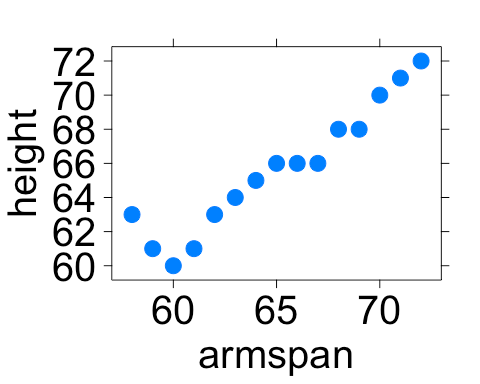# LAB 4C: Cross-Validation

## Lab 4C - Cross-Validation

### Predictions

• In the previous lab, we learned how to calculate the mean squared error (MSE).

– This let us measure how well our model predicts values of our `y`-variable.

• To really measure how well our line of best fit predicts people's `heights`, we want see how well we predict the `heights` of people that we haven't yet measured.

• To do this, we'll divide our data into two sets:

– A training set used to build our model.

– And a testing set we can use to measure how well our model predicts new data values.

• This method of dividing data into sets is called cross-validation.

### Why cross-validate?

• Data scientists are often tasked with predicting some aspect of future observations.

– Relying on a single data set to both train and test models can lead to models that are so specific to the current batch of data that they're unable to make good predictions for these future observations.

• Cross-validating allows data scientists to measure how well their models predict new observations.

– It also gives them the ability to compare different models to see which models make better/worse predictions.

### Splitting the data

• Waiting for new observations can take a long time. The U.S. takes a census of its population once every 10 years, for example.

• Instead of waiting for new observations, data scientists will take their current data and divide it into two distinct sets.

• For our `arm_span` data, fill in the blanks to create a `training` and `testing` data set.

``````set.seed(123)
train_rows <- sample(1:____, size = 85)
train <- slice(arm_span, ____)
test <- slice(____, - ____)
``````
• Explain these lines of code and describe the `train` and `test` data sets.

### set.seed then split

• When we split data, we're randomly separating our observations into training and testing sets.

– It's important to notice that no single observation will be placed in both sets.

• Because we're splitting the data sets randomly, our models can will also vary slightly, person-to-person.

– This is why it's important to use `set.seed`.

• By using `set.seed`, we're able to reproduce the random splitting so that each person's model outputs the same results.

Whenever you split data into training and testing, always use `set.seed` first.

### Building on training

• When splitting data into training and testing sets, we need to have enough observations in our data so that we can build a good model.

– This is why we kept 85 observations in our `training` data.

• As data sets grow larger, we can use a larger proportion of the data to test with.

• Fit a line of best fit model to our training data and assign it the name `best_train`.

### Predicting on testing

• Now that our model has been built, we can use it to predict the values of `height` in our `test` data.

• Because we're using the line of best fit, we can use the `predict()` function we introduced in the last lab to make predictions.

– Fill in the blanks below to add predicted heights to our test data:

``````test <- mutate(test, ____ = predict(best_train, newdata = ____))
``````
• Calculate the MSE in the same way as you did in the previous lab.

### Avoiding being too specific

• When we build models without cross-validating, we run the risk of building models that are too specific to the data we already have.

– Meaning, the model predicts values we know about really well BUT predicts new values very poorly.

• The plot on the following slide shows a single, randomly chosen `height` for each value of `armspan`.• With a neighbor, write down a prediction rule that would predict a person's `height` based on their `armspan` really well for people already shown in our plot but would predict people not in our plot very poorly.